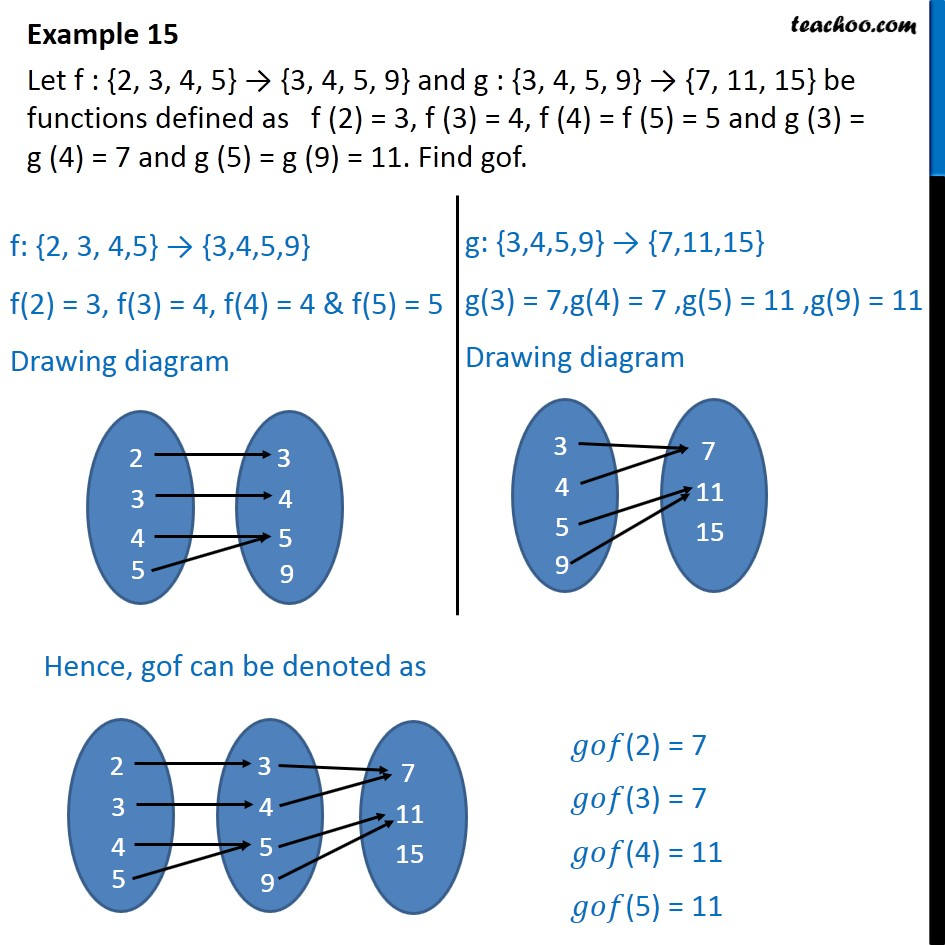Composite functions

Chapter 1 Class 12 Relation and Functions
Concept wiseIntroducing your new favourite teacher - Teachoo Black, at only ₹83 per month

### Transcript

Example 15 Let f : {2, 3, 4, 5} → {3, 4, 5, 9} and g : {3, 4, 5, 9} → {7, 11, 15} be functions defined as f (2) = 3, f (3) = 4, f (4) = f (5) = 5 and g (3) = g (4) = 7 and g (5) = g (9) = 11. Find gof.